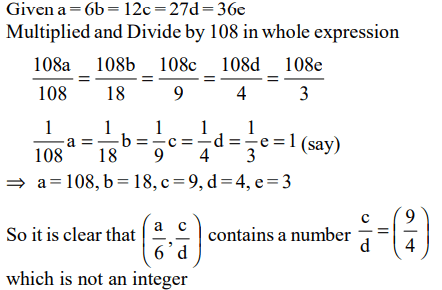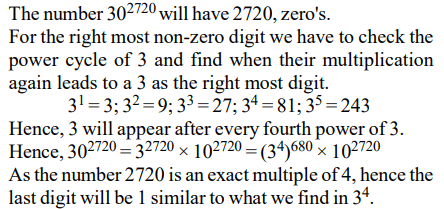## Number System Previous Year CAT Questions

1. Let a, b, c, d and e be integers such that a = 6b = 12c, and 2b = 9d = 12e. Then which of the following pairs contains a number that is not an integer?
a) $\left(\frac{a}{27},\frac{b}{e}\right)$
b) $\left(\frac{a}{36},\frac{c}{e}\right)$
c) $\left(\frac{a}{12},\frac{bd}{18}\right)$
d) $\left(\frac{a}{6},\frac{c}{d}\right)$

Explanation:2. If a, a + 2 and a + 4 are prime numbers, then the number of possible solutions for a is
a) one
b) two
c) three
d) more than three

Explanation: a, a + 2, a + 4 are prime numbers.
Put value of ‘a’ starting from 3, we will have 3, 5 and 7 as the only set of prime numbers satisfying the given relationships

3. The remainder, when $\left(15^{23}+23^{23}\right)$   is divided by 19, is
a) 4
b) 15
c) 0
d) 14

Explanation: The expression becomes (19 – 4)23 + (19 + 4)23
All the terms except the last one contains 19 and the last terms get cancelled out. Hence the remainder obtained on dividing by 19 will be 0

4. If $x=\left(16^{3}+17^{3}+18^{3}+19^{3}\right)$     , then x divided by 70 leaves a remainder of
a) 0
b) 1
c) 69
d) 35

Explanation:5. The digits of a three-digit number A are written in the reverse order to form another three-digit number B. If B > A and B–A is perfectly divisible by 7, then which of the following is necessarily true?
a) 100 < A < 299
b) 106 < A < 305
c) 112 < A < 311
d) 118 < A < 317

Explanation: Let the 3 digits of number A be x, y and z
Hence A = 100x + 10y + z
On reversing the digits of number A, we get the number
B i.e., z y x.
B = 100z + 10y + x6. The rightmost non-zero digit of the number $30^{2720}$ is
a) 1
b) 3
c) 7
d) 9

Explanation:7. If $\frac{30^{65}-29^{65}}{30^{64}+29^{64}}$  then
a) $0< R\leq 0.1$
b) $0.1< R\leq 0.5$
c) $0.5< R\leq 1.0$
d) R > 1.0

Explanation:8. For a positive integer n, let $p_{n}$ denote the product of the digits of n, and $s_{n}$ denote the sum of the digits of n. The number of integers between 10 and 1000 for which $p_{n}+s_{n}=n$ is
a) 81
b) 16
c) 18
d) 9

Explanation:9. If x = –0.5, then which of the following has the smallest value?
a) $2^{\frac{1}{x}}$
b) $\frac{1}{x}$
c) $\frac{1}{x^{2}}$
d) $2^{x}$10. Which one among $2^{1/2},3^{1/3},4^{1/4},6^{1/6}$   and $12^{1/12}$ is the largest?
a) $2^{1/2}$
b) $3^{1/3}$
c) $4^{1/4}$
d) $6^{1/6}$